# Math in Focus Grade 3 Chapter 19 Practice 4 Answer Key Area and Perimeter

This handy Math in Focus Grade 3 Workbook Answer Key Chapter 19 Practice 4 Area and Perimeter detailed solutions for the textbook questions.

## Math in Focus Grade 3 Chapter 19 Practice 4 Answer Key Area and Perimeter

Complete. Find the perimeter and area of each shaded figure.

Question 1.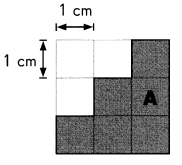Perimeter of Figure A is __________ centimeters.
Area of Figure A is __________ square centimeters.
Perimeter of Figure A is 12 cm centimeters.
Area of Figure A is 6 square centimeters.
Explanation:
Perimeter is the distance around the edge of a shape.
Area is used to define the amount of space taken up by a 2D shape or surface.
We measure area in square units : cm² or m².
Area is calculated by multiplying the length of a shape by its width.

Question 2.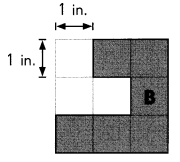Perimeter of Figure B is __________ inches.
Area of Figure B is __________ square inches.
Perimeter of Figure B is 13 inches.
Area of Figure B is 6 square inches.
Explanation:
Perimeter is the distance around the edge of a shape.
Area is used to define the amount of space taken up by a 2D shape or surface.
We measure area in square units : cm² or m².
Area is calculated by multiplying the length of a shape by its width.

Question 3.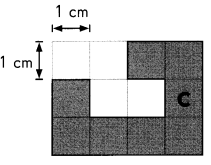Perimeter of Figure C is __________ centimeters.
Area of Figure C is __________ square centimeters.
Perimeter of Figure C is 18 centimeters.
Area of Figure C is 8 square centimeters.
Explanation:
Perimeter is the distance around the edge of a shape.
Area is used to define the amount of space taken up by a 2D shape or surface.
We measure area in square units : cm² or m².
Area is calculated by multiplying the length of a shape by its width.

Question 4.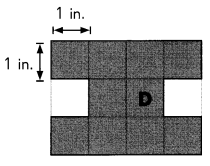Perimeter of Figure D is __________ inches.
Area of Figure D is __________ square inches.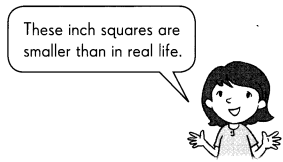Perimeter of Figure D is 18 inches.
Area of Figure D is 10 square inches.
Explanation:
The perimeter of a two-dimensional figure is the distance covered around it.
It defines the length of shape, whether it is a triangle, square, rectangle or a circle.
Area is the number of unit squares equal in measure to the surface of a figure.

Complete. Find the perimeter and area of each shaded figure.

Question 5.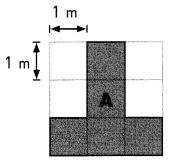Perimeter of Figure A is __________ meters.
Area of Figure A is __________ square meters.
Perimeter of Figure A is 12 meters.
Area of Figure A is 5 square meters.
Explanation:
Perimeter is the distance around the edge of a shape.
Area is used to define the amount of space taken up by a 2D shape or surface.
We measure area in square units : cm² or m².
Area is calculated by multiplying the length of a shape by its width.

Question 6.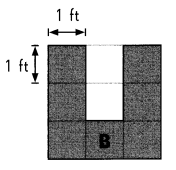Perimeter of Figure B is __________ feet.
Area of Figure B is __________ square feet.
Perimeter of Figure B is 16 feet.
Area of Figure B is 7 square feet.
Explanation:
Perimeter is the distance around the edge of a shape.
Area is used to define the amount of space taken up by a 2D shape or surface.
We measure area in square units : cm² or m².
Area is calculated by multiplying the length of a shape by its width.

Question 7.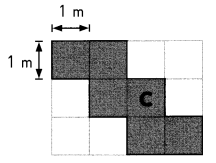Perimeter of Figure C is __________ meters.
Area of Figure C is __________ square meters.
Perimeter of Figure C is 14 meters.
Area of Figure C is 6 square meters.
Explanation:
Perimeter is the distance around the edge of a shape.
Area is used to define the amount of space taken up by a 2D shape or surface.
We measure area in square units : cm² or m².
Area is calculated by multiplying the length of a shape by its width.

Question 8.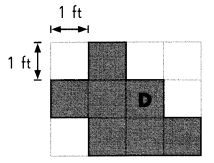Perimeter of Figure D is __________ feet.
Area of Figure D is __________ square feet.
Perimeter of Figure D is 14 feet.
Area of Figure D is 7 square feet.
Explanation:
Perimeter is the distance around the edge of a shape.
Area is used to define the amount of space taken up by a 2D shape or surface.
We measure area in square units : cm² or m².
Area is calculated by multiplying the length of a shape by its width.

Draw two different figures with an area of 5 square centimeters.

Question 9.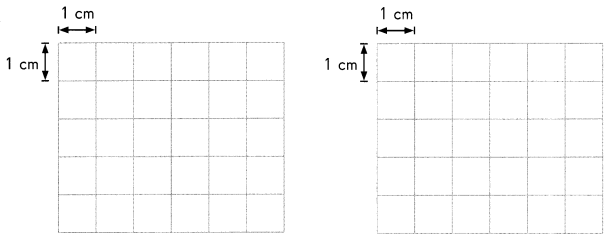Do they have the same perimeter? _____________
Explanation:
Both have different perimeter,
As, the perimeter of a two-dimensional figure is the distance covered around it.
It defines the length of shape, whether it is a triangle, square, rectangle, circle and so on.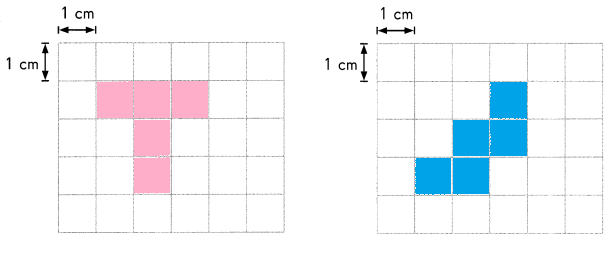Draw two different figures with a perimeter of 8 centimeters.

Question 10.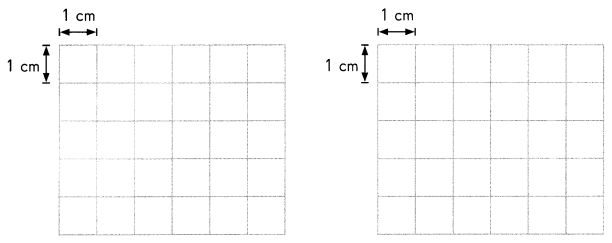Do they have the same area? _____________
yes, they have the same area.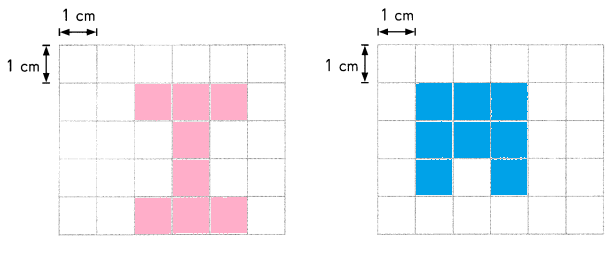Explanation:
Area is the amount of space taken up by a 2D shape or surface.
We measure area in square units : cm² or m².
Area is calculated by multiplying the length of a shape by its width.

Find the perimeter and area of each figure.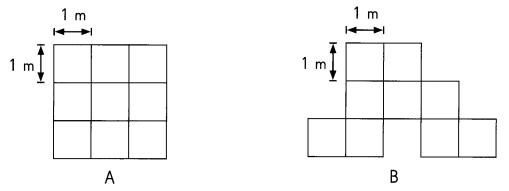Question 11.
Perimeter = ___________
Area = __________
Perimeter = 12 m
Area =9 m2
Explanation:
Perimeter is the distance around the edge of a shape.
Area is used to define the amount of space taken up by a 2D shape or surface.
We measure area in square units : cm² or m².
Area is calculated by multiplying the length of a shape by its width.

Question 12.
Perimeter = _____________
Area = ___________
Perimeter = 15m
Area = 9 m2
Explanation:
Perimeter is the distance around the edge of a shape.
Area is used to define the amount of space taken up by a 2D shape or surface.
We measure area in square units : cm² or m².
Area is calculated by multiplying the length of a shape by its width.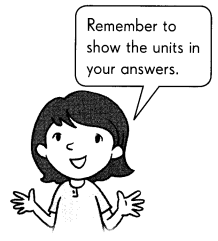Write yes or no.

Question 13.
Do Figures A and B have the same area? ___________
Explanation:
Area is the amount of space taken up by a 2D shape or surface.
Figure A and B have the same are
just by counting the 1×1 m box areas in the given figure will be the area of the figure.

Question 14.
Do Figures A and B have the same perimeter? ___________
Explanation:
Perimeter is the distance around the edge of a shape.
Figure A and B do not have the same perimeter.
the shape of the figure A and B are different in shape,
the perimeters are also different to each other.

Question 15.
What is different about perimeter and area? Explain.